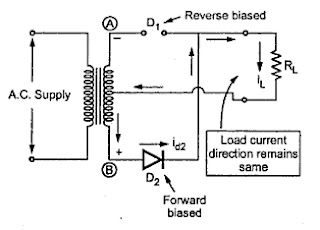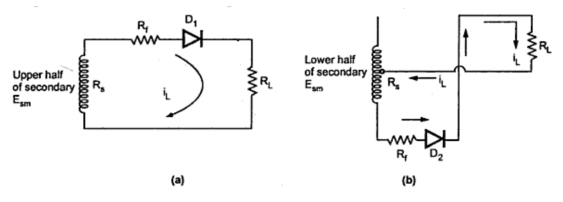### Full Wave Recftifier : Part1

The full wave rectifier conducts during both positive and negative half cycles of input a.c. supply. In order to rectify both the half cycles of a.c. input, two diodes are used in this circuit. The diodes feed a common load RL with the help of a center tap transformer. The a.c. voltage is applied through a suitable power transformer with proper turns ratio.
The full wave rectifier circuit is shown in the Fig. 1.Fig. 1  Full wave rectifier

For the proper operation of the circuit, a center-tap on the secondary winding of the transformer is essential.
1.1 Operation of the Circuit
Consider the positive half cycle of a.c. input voltage in which terminal (A) is positive and terminal (B) negative. The diode D1 will be forward biased and hence will conduct; while diode D2 will be reverse biased and will act as an open circuit and will not conduct. This is illustrated in the Fig. 2.Fig. 2  Current flow during positive half cycle

The diode D1 supplies the load current, i.e. IL = Id2. This current is flowing through upper half of secondary winding of the transformer carries no current since diode D2 is reverse biased and acts as an open circuit.
In the next half cycle of a.c. voltage, polarity reverses and terminal (A) becomes negative and (B) positive. The diode D2 conducts, being forward biased, while D1 does not, being reverse biased. This is shown in the Fig. 3.Fig. 3  Current flow during negative half cycle

The diode D2 supplies the load current, i.e. IL = Id2. Now the lower half of the secondary winding carries the current but the upper half does not.
Note : It is noted that the load current flows in both the half cycles of a.c. voltage and in the same direction through the load resistance.
Hence we get rectified output across the load. The load current is sum of individual diode currents flowing in corresponding half cycles. It is also noted that the two diodes do not conduct simultaneously but in alternate half cycles. The individual diode currents and the load current are shown in the Fig. 4.Fig. 4  Load current and voltage waveforms for full wave rectifier

Thus the full wave rectifier circuit essentially consists of two half-wave rectifier circuits working independently (working in alternate half cycles of a.c.) of each other but feeding a common load. The output load current is still pulsating d.c. and not pure d.c.
Let    Rf  = Forward resistance of diodes
Rs = Winding resistance of each half of secondary
es = Instantaneous a.c. voltage across each half of secondary
...      es = Esm sin ωt
ω = 2 π f
Esm = Maximum value of a.c. input voltage across each half of secondary winding
Hence we can write the expression for the maximum value of the load current, looking at equivalent circuit shown in the Fig. 5.Fig. 5
where Im = Maximum value of load current IL.
1.3 Average D.C. Load Current (IDC)
Consider one cycle of load current IL from 0 to 2π to obtain the average value of load current.Fig. 6

IL = Im sinωt           0≤ ωt≤ π
But for π to 2π, the current is again positive while sint term is negative during π to 2π. Hence in the region π to 2π the positive IL can be represented as negative of Im sin (ωt).
...          IL = -Im sin ωt                    π ≤ ωt ≤ 2π
But cosπ = -1
For half wave it is Im/π and full wave rectifier is the combination of two half wave circuits acting alternately in two half cycles of input. Hence obviously the d.c. value for full wave circuits 2Im/π.
1.4 Average D.C. Load Voltage (EDC)